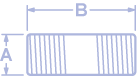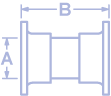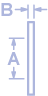## Flo-Controlet

Flo-Controlet is an established line of fully automatic metering flow controllers made in the U.S. These reliable products maintain a constant liquid flow with changes of inlet pressure from 30 to 120 psi. They are designed for commercial/industrial applications including water softeners, filters, cooling towers, irrigation, industry, recreation, etc. Models are constructed of PVC, steel, or brass, and are available in a variety of configurations to provide flow rates from 0.25 to 540 gpm.

# Flo-Controlet Products

•• OM-TH0501/(FR)
• Pipe Size “A”: 1/2 inch
• Number of Inserts: 1
• (FR) Flow Rate: 0.25 to 3.5 gpm
• Length “B”: 2 inch
• Weight Approx: 0.05 lb
• OM-TH0751/(FR)
• Pipe Size “A”: 3/4 inch
• Number of Inserts: 1
• (FR) Flow Rate: 0.25 to 10 gpm
• Length “B”: 2 inch
• Weight Approx: 0.06 lb
• OM-TH1001/(FR)
• Pipe Size “A”: 1 inch
• Number of Inserts: 1
• (FR) Flow Rate: 5 to 10 gpm
• Length “B”: 3 inch
• Weight Approx: 0.12 lb
• OM-TH1001C/(FR)
• Pipe Size “A”: 1 inch
• Number of Inserts: 1
• (FR) Flow Rate: 12, 15, 20 gpm
• Length “B”: 3 inch
• Weight Approx: 0.12 lb
• OM-TH1252/(FR)
• Pipe Size “A”: 1-1/4 inch
• Number of Inserts: 2
• (FR) Flow Rate: 5 to 20 gpm
• Length “B”: 3 inch
• Weight Approx: 0.18 lb
• OM-TH1504/(FR)
• Pipe Size “A”: 1-1/2 inch
• Number of Inserts: 4
• (FR) Flow Rate: 5 to 40 gpm
• Length “B”: 3 inch
• Weight Approx: 0.25 lb
• OM-TH2007/(FR)
• Pipe Size “A”: 2 inch
• Number of Inserts: 7
• (FR) Flow Rate: 5 to 70 gpm
• Length “B”: 3 inch
• Weight Approx: 0.31 lb
• OM-TH2509/(FR)
• Pipe Size “A”: 2-1/2 inch
• Number of Inserts: 9
• (FR) Flow Rate: 5 to 90 gpm
• Length “B”: 4 inch
• Weight Approx: 0.56 lb
• OM-TH30014/(FR)
• Pipe Size “A”: 3 inch
• Number of Inserts: 14
• (FR) Flow Rate: 5 to 140 gpm
• Length “B”: 4 inch
• Weight Approx: 0.68 lb
• OM-TH40025/(FR)
• Pipe Size “A”: 4 inch
• Number of Inserts: 25
• (FR) Flow Rate: 5 to 250 gpm
• Length “B”: 4 inch
• Weight Approx: 1.00 lb
• OM-TH60054/(FR)
• Pipe Size “A”: 6 inch
• Number of Inserts: 54
• (FR) Flow Rate: 5 to 540 gpm
• Length “B”: 5 inch
• Weight Approx: 2.50 lb
• PVC Spigot
•• OM-PE0501/(FR)
• Pipe Size “A”: 1/2 inch
• Number of Inserts: 1
• (FR) Flow Rate: 0.25 to 3.5 gpm
• Length “B”: 5 inch
• Weight Approx: 0.09 lb
• OM-PE0751/(FR)
• Pipe Size “A”: 3/4 inch
• Number of Inserts: 1
• (FR) Flow Rate: 0.25 to 10 gpm
• Length “B”: 5 inch
• Weight Approx: 0.12 lb
• OM-PE1001/(FR)
• Pipe Size “A”: 1 inch
• Number of Inserts: 1
• (FR) Flow Rate: 5 to 10 gpm
• Length “B”: 6 inch
• Weight Approx: 0.18 lb
• OM-PE1001C/(FR)
• Pipe Size “A”: 1 inch
• Number of Inserts: 1
• (FR) Flow Rate: 12, 15, 20 gpm
• Length “B”: 6 inch
• Weight Approx: 0.18 lb
• OM-PE1252/(FR)
• Pipe Size “A”: 1-1/4 inch
• Number of Inserts: 2
• (FR) Flow Rate: 5 to 20 gpm
• Length “B”: 6 inch
• Weight Approx: 0.27 lb
• OM-PE1504/(FR)
• Pipe Size “A”: 1-1/2 inch
• Number of Inserts: 4
• (FR) Flow Rate: 5 to 40 gpm
• Length “B”: 6 inch
• Weight Approx: 0.36 lb
• OM-PE2007/(FR)
• Pipe Size “A”: 2 inch
• Number of Inserts: 7
• (FR) Flow Rate: 5 to 70 gpm
• Length “B”: 6 inch
• Weight Approx: 0.47 lb
• OM-PE2509/(FR)
• Pipe Size “A”: 2-1/2 inch
• Number of Inserts: 9
• (FR) Flow Rate: 5 to 90 gpm
• Length “B”: 6 inch
• Weight Approx: 0.80 lb
• OM-PE30014/(FR)
• Pipe Size “A”: 3 inch
• Number of Inserts: 14
• (FR) Flow Rate: 5 to 140 gpm
• Length “B”: 6 inch
• Weight Approx: 1.00 lb
• OM-PE40025/(FR)
• Pipe Size “A”: 4 inch
• Number of Inserts: 25
• (FR) Flow Rate: 5 to 250 gpm
• Length “B”: 6 inch
• Weight Approx: 1.47 lb
• OM-PE60054/(FR)
• Pipe Size “A”: 6 inch
• Number of Inserts: 54
• (FR) Flow Rate: 5 to 540 gpm
• Length “B”: 8 inch
• Weight Approx: 3.90 lb
• PVC ANSI Flanged
•• OM-FL0501/(FR)
• Pipe Size “A”: 1/2 inch
• Number of Inserts: 1
• (FR) Flow Rate: 0.25 to 3.5 gpm
• Length “B”: 5 inch
• Weight Approx: 0.49 lb
• OM-FL0751/(FR)
• Pipe Size “A”: 3/4 inch
• Number of Inserts: 1
• (FR) Flow Rate: 0.25 to 10 gpm
• Length “B”: 5 inch
• Weight Approx: 0.68 lb
• OM-FL1001/(FR)
• Pipe Size “A”: 1 inch
• Number of Inserts: 1
• (FR) Flow Rate: 5 to 10 gpm
• Length “B”: 6 inch
• Weight Approx: 0.96 lb
• OM-FL1001C/(FR)
• Pipe Size “A”: 1 inch
• Number of Inserts: 1
• (FR) Flow Rate: 12, 15, 20 gpm
• Length “B”: 6 inch
• Weight Approx: 0.96 lb
• OM-FL1252/(FR)
• Pipe Size “A”: 1-1/4 inch
• Number of Inserts: 2
• (FR) Flow Rate: 5 to 20 gpm
• Length “B”: 6 inch
• Weight Approx: 1.27 lb
• OM-FL1504/(FR)
• Pipe Size “A”: 1-1/2 inch
• Number of Inserts: 4
• (FR) Flow Rate: 5 to 40 gpm
• Length “B”: 6 inch
• Weight Approx: 1.64 lb
• OM-FL2007/(FR)
• Pipe Size “A”: 2 inch
• Number of Inserts: 7
• (FR) Flow Rate: 5 to 70 gpm
• Length “B”: 6 inch
• Weight Approx: 2.43 lb
• OM-FL2509/(FR)
• Pipe Size “A”: 2-1/2 inch
• Number of Inserts: 9
• (FR) Flow Rate: 5 to 90 gpm
• Length “B”: 6 inch
• Weight Approx: 3.80 lb
• OM-FL30014/(FR)
• Pipe Size “A”: 3 inch
• Number of Inserts: 14
• (FR) Flow Rate: 5 to 140 gpm
• Length “B”: 6 inch
• Weight Approx: 4.76 lb
• OM-FL40025/(FR)
• Pipe Size “A”: 4 inch
• Number of Inserts: 25
• (FR) Flow Rate: 5 to 250 gpm
• Length “B”: 6 inch
• Weight Approx: 7.55 lb
• OM-FL60054/(FR)
• Pipe Size “A”: 6 inch
• Number of Inserts: 54
• (FR) Flow Rate: 5 to 540 gpm
• Length “B”: 8.5 inch
• Weight Approx: 12.60 lb
• PVC ANSI Flanged Disc
•• OM-DI2007/(FR)
• Pipe Size “A”: 2 inch
• Number of Inserts: 7
• (FR) Flow Rate: 5 to 70 gpm
• Length “B”: 15/16 inch
• Weight Approx: 1.12 lb
• OM-DI2509/(FR)
• Pipe Size “A”: 2-1/2 inch
• Number of Inserts: 9
• (FR) Flow Rate: 5 to 90 gpm
• Length “B”: 15/16 inch
• Weight Approx: 1.50 lb
• OM-DI30014/(FR)
• Pipe Size “A”: 3 inch
• Number of Inserts: 14
• (FR) Flow Rate: 5 to 140 gpm
• Length “B”: 15/16 inch
• Weight Approx: 1.75 lb
• OM-DI40025/(FR)
• Pipe Size “A”: 4 inch
• Number of Inserts: 25
• (FR) Flow Rate: 5 to 250 gpm
• Length “B”: 15/16 inch
• Weight Approx: 2.48 lb
• OM-DI60054/(FR)
• Pipe Size “A”: 6 inch
• Number of Inserts: 54
• (FR) Flow Rate: 5 to 540 gpm
• Length “B”: 15/16 inch
• Weight Approx: 3.73 lb
•• OM-ST0751/(FR)
• Pipe Size “A”: 0.75 inch
• Number of Inserts: 1
• (FR) Flow Rate: 0.25 to 10 gpm
• Length “B”: 2-1/2 inch
• Weight Approx: 0.28 lb
• OM-ST1001/(FR)
• Pipe Size “A”: 1 inch
• Number of Inserts: 1
• (FR) Flow Rate: 5 to 10 gpm
• Length “B”: 3 inch
• Weight Approx: 0.50 lb
• OM-ST1001C/(FR)
• Pipe Size “A”: 1 inch
• Number of Inserts: 1
• (FR) Flow Rate: 12, 15, 20 gpm
• Length “B”: 3 inch
• Weight Approx: 0.53 lb
• OM-ST1252/(FR)
• Pipe Size “A”: 1-1/4 inch
• Number of Inserts: 2
• (FR) Flow Rate: 5 to 20 gpm
• Length “B”: 3 inch
• Weight Approx: 0.62 lb
• OM-ST1504/(FR)
• Pipe Size “A”: 1-1/2 inch
• Number of Inserts: 4
• (FR) Flow Rate: 5 to 40 gpm
• Length “B”: 3 inch
• Weight Approx: 0.78 lb
• OM-ST2007/(FR)
• Pipe Size “A”: 2 inch
• Number of Inserts: 7
• (FR) Flow Rate: 5 to 70 gpm
• Length “B”: 3 inch
• Weight Approx: 1.06 lb
• OM-ST2509/(FR)
• Pipe Size “A”: 2-1/2 inch
• Number of Inserts: 9
• (FR) Flow Rate: 5 to 90 gpm
• Length “B”: 4 inch
• Weight Approx: 2.15 lb
• OM-ST30014/(FR)
• Pipe Size “A”: 3 inch
• Number of Inserts: 14
• (FR) Flow Rate: 5 to 140 gpm
• Length “B”: 4 inch
• Weight Approx: 2.75 lb
• OM-ST40025/(FR)
• Pipe Size “A”: 4 inch
• Number of Inserts: 25
• (FR) Flow Rate: 5 to 250 gpm
• Length “B”: 4 inch
• Weight Approx: 4.09 lb
• OM-ST60054/(FR)
• Pipe Size “A”: 6 inch
• Number of Inserts: 54
• (FR) Flow Rate: 5 to 540 gpm
• Length “B”: 5 inch
• Weight Approx: 7.85 lb
•• OM-BR10501/(FR)
• Pipe Size “A”: 1/2 inch
• Number of Inserts: 1
• (FR) Flow Rate: 0.25 to 3.5 gpm
• Length “B”: 2 inch
• Weight Approx: 0.12 lb
• OM-BR20501/(FR)
• Pipe Size “A”: 1/2 inch
• Number of Inserts: 1
• (FR) Flow Rate: 5 to 10 gpm
• Length “B”: 2 inch
• Weight Approx: 0.12 lb

# Contact CIE

CIE, Inc.
996 Bluff City Boulevard
Elgin, IL 60120 USA

Tel: 847-888-8338
Fax: 847-888-6924
E-mail: sales@cie-inc.com